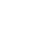### Box Compression Test Calculator: BCT Strength Calculator

Estimates the BCT of a regular slotted container (RSC) for a moment with flutes positioned vertically. (Note that BCT is not stacking strength, which is the maximum load a box can handle throughout the distribution cycle.)

\begin{array}{l}{\text {Box Compression Test }(\mathrm{BCT})=\mathrm{k}_{1} * \mathrm{ECT} \times \sqrt{\mathrm{h} * Z}} \\\\ {\text {Reference Mckee formula (simplified version) }} \\ { { Where: }} \\ { \mathrm{k} 1=\mathrm{a} \text { constant value of } 5.87} \\ {\mathrm{h}=\text { Corrugated FiberBoard (CFB) thickness (inches) }} \\ {\mathrm{Z}=\text { box perimeter (inches) = } 2(\mathrm{L}+\mathrm{W})}\end{array}Box Compression Test (BCT) Strength Calculator

Invalid Input

Pounds / Inches

Invalid Input

Inches

Invalid Input

Inches

Invalid Input

Inches

Calculate
Thanks for using our services!
Enter some brief information to see results
• We will send you newsletters that you can opt-out for at anytime. We do not share your information.
• Hidden
See ResultsBox Compression Test (BCT) Strength CalculatorEdge Crush Test (ECT):
Corrugated board thickness (h):
Box length (L):
Box width (W):
Box Compression Test (BCT):
Reset
NOTES
1. English units are used because CT Values are typically in pounds per inch units.
2. The simplified McKee formula above provides a quick estimate that should not be used to rate the strength of an actual box. Such an estimate shall not to be used in place of testing conditioned test samples in a controlled, repeatable test environment, with calibrated instrumentation, validated machines, and trained test operators.
3. The ECT Value is often provided on the BMC (Box Manufacturer’s Certificate).If this data is not provided by your supplier, Westpak can provide testing to determine or verify this value.Box Compression Test (BCT) Strength Calculator

Invalid Input

Pounds / Inches

Invalid Input

Inches

Invalid Input

Inches

Invalid Input

Inches

Calculate
Thanks for using our services!
Enter some brief information to see results
• We will send you newsletters that you can opt-out for at anytime. We do not share your information.
• Hidden
See ResultsBox Compression Test (BCT) Strength CalculatorEdge Crush Test (ECT):
Corrugated board thickness (h):
Box length (L):
Box width (W):
Box Compression Test (BCT):
Reset